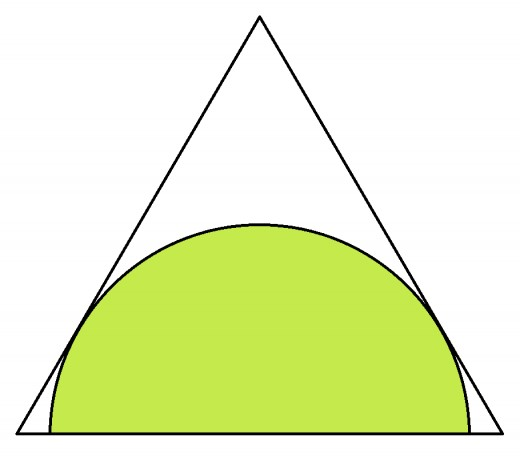# SOLVE THIS !

Geometry Level 2A half-circle is inscribed within an equilateral triangle such that the diameter of the half-circle is centered on one edge of the triangle and the arc is tangent to the other two sides. What is the diameter of the semi-circle if the triangle has an edge length of 4?

×